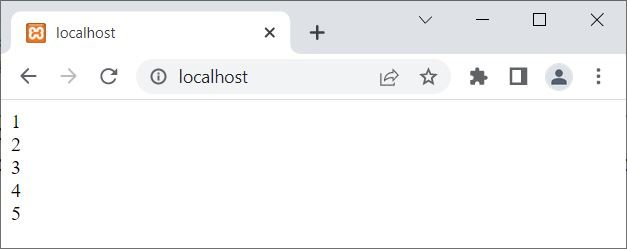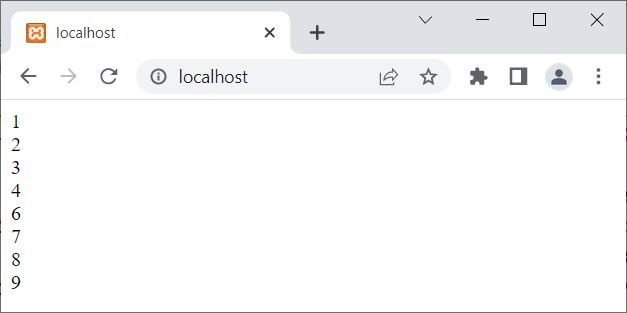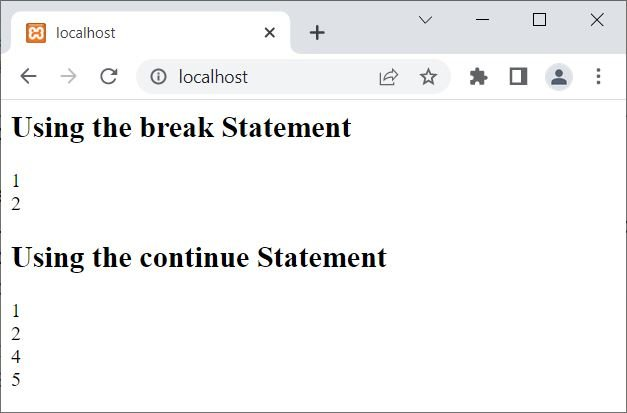# break and continue Statement in PHP

## PHP break statement

The PHP break keyword or statement is used when we need to exit from the current loop or switch structure. For example:

```<?php
for(\$i=1; \$i<10; \$i++)
{
if(\$i>5)
break;

echo \$i;
echo "<BR>";
}
?>```

The snapshot given below shows the output produced by this PHP example on the break statement:In the above example, when the value of \$i becomes greater than 5, that is, when the value of \$i becomes 6, then the condition \$i>5 or 6>5 evaluates to be true, and therefore, the program flow goes inside the if block and the "break;" statement gets executed, which skips the remaining execution of the current for loop. Therefore, we only get the output from 1 to 5.

The break statement skips the execution of the remaining statement(s) (if any) along with all the remaining iterations of the current loop.

The break statement can also be used to stop the execution of an infinite loop (the loop whose condition always evaluates to true). For example:

```<?php
\$num = 10;
while(true)
{
echo \$num, "<BR>";

if(\$num==12)
break;

\$num++;
echo \$num, "<BR>";
}
?>```

The output of this PHP example should be:See the condition of the loop; it is true, which obviously evaluates to being true forever. Therefore, using the break statement, the execution of this loop ends.

The following is the dry run of the preceding example:

• The value 10 was initialized to the variable \$num using the first statement.
• Now the execution of the while loop has started.
• Since the condition of the loop is true, therefore, the loop goes for its infinite execution.
• The value of \$num is printed along with a line break using the BR tag in the first iteration using echo.
• Since the condition \$num==12 or 10==12 evaluates to false, program flow will not go inside the if block to execute the break statement.
• The value of \$num gets incremented by 1. Now \$num=11.
• The value of \$num again gets printed along with a line break.
• Now the second iteration of the loop starts.
• This process continues until the value of \$num becomes 12.
• When the value of \$num becomes 12, the condition \$num==12 (of if) evaluates to true, and the break; statement gets executed, which forces the while loop to stop its execution.

## PHP continue statement

The PHP continue statement or keyword is used when we need to skip the execution of remaining statement(s) for the current iteration of the current loop and continue for the next iteration of the current loop. For example:

```<?php
for(\$i=1; \$i<10; \$i++)
{
if(\$i==5)
continue;

echo \$i;
echo "<BR>";
}
?>```

The output of the above PHP example on the continue statement is:In the above example, when the value of \$i becomes 5, the condition \$i==5 evaluates to be true. Therefore, the continue statement gets executed, which forces the program to jump and continue the execution of the next iteration of the current loop. That is, the two echo statements get skipped for that iteration.

## Difference between break and continue in PHP

The break is used to skip all the remaining executions and iterations of the current loop. where the continue keyword is used to skip the execution of the remaining statement(s) of the current iteration of the current loop. For example:

```<?php
echo "<h2>Using the break Statement</h2>";
for(\$i=1; \$i<=5; \$i++)
{
if(\$i==3)
break;
echo \$i, "<BR>";
}

echo "<h2>Using the continue Statement</h2>";
for(\$i=1; \$i<=5; \$i++)
{
if(\$i==3)
continue;
echo \$i, "<BR>";
}
?>```

The output of the above PHP example is:PHP Online Test

« Previous Tutorial Next Tutorial »